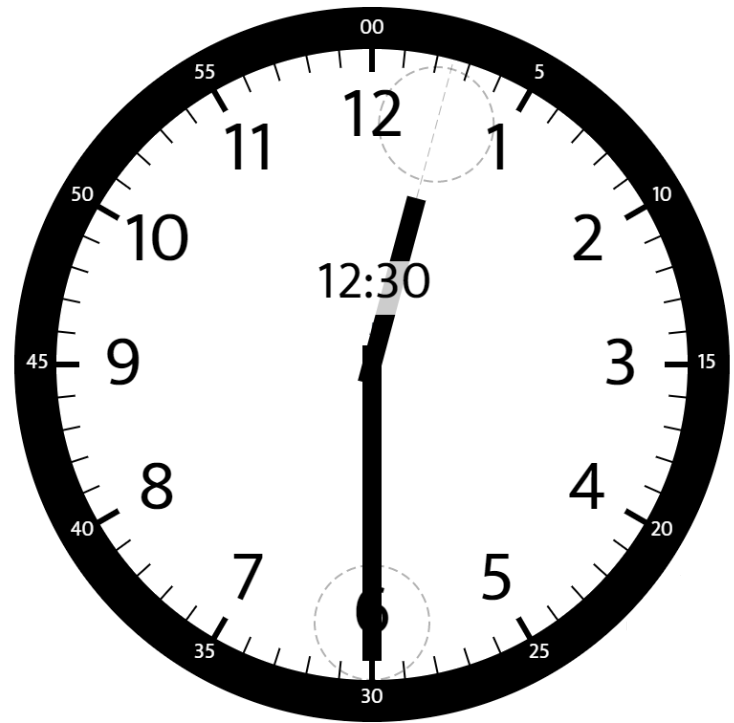# Leetcode BiWeekly Contest 19 题解

## 1342. Number of Steps to Reduce a Number to Zer

Given a non-negative integer num, return the number of steps to reduce it to zero. If the current number is even, you have to divide it by 2, otherwise, you have to subtract 1 from it.

## Number of Sub-arrays of Size K and Average Greater than or Equal to Threshold

Given an array of integers arr and two integers k and threshold.
Return the number of sub-arrays of size k and average greater than or equal to threshold.

### 1344. Angle Between Hands of a Clock

Given two numbers, hour and minutes. Return the smaller angle (in sexagesimal units) formed between the hour and the minute hand.1. 普通钟表相当于圆，其时针或分针走一圈均相当于走过360°角；

2. 钟表上的每一个大格（时针的一小时或分针的5分钟）对应的角度是：360°/12=30°；

3. 时针每走过1分钟对应的角度应为：360°/(12*60)=0.5°；

4. 分针每走过1分钟对应的角度应为：360°/60=6°。

## 1345. Jump Game IV

Given an array of integers arr, you are initially positioned at the first index of the array.
In one step you can jump from index i to index:

1. i + 1 where: i + 1 < arr.length.
2. i - 1 where: i - 1 >= 0.
3. j where: arr[i] == arr[j] and i != j.
Return the minimum number of steps to reach the last index of the array.
Notice that you can not jump outside of the array at any time.

1. 在 i+1 或 i-1 都在数组的范围内

2. 如果存在 index=j 且 arr[i]==arr[j] 且 i!=j 的时候，可以直接从 i 跳到 j

1. 构建一个字典，值为key，index 为 value（相同的值之间可以直接跳）

2. 利用一个 set 来保存跳过的点

3. 从 index = 0 开始进行 BFS ，求每个点在一步之内可以跳到哪个点，然后不断的 BFS 直到到达终点

4. 更新被访问过的点

emmmm，好吧 BFS ，开写吧

Manjusaka

2020-02-11

2020-11-26